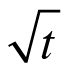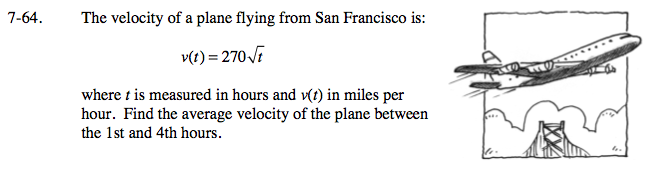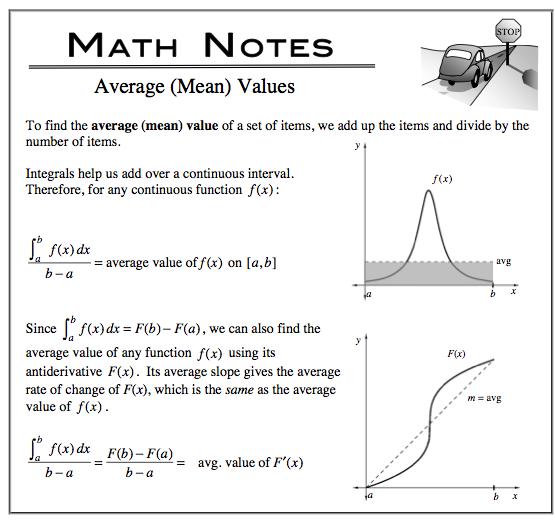### Home > CALC > Chapter 7 > Lesson 7.2.1 > Problem7-64

7-64.
1. The velocity of a plane flying from San Francisco is:

v(t) = 2702. where t is measured in hours and v(t) in miles per hour. Find the average velocity of the plane between the 1st and 4th hours. Homework Help ✎You are given a velocity function and you want to find average velocity.
Should you use 'average area under the curve' or 'slope of the secant'?